CA168首页 > 自动化新闻 > 产品新闻 > 新闻详情

EN600变频器助力冷弯型钢机组2018年10月23日一座跨越伶仃洋，东接香港，西接广东珠海和澳门，总长约55公里，由粤港澳三地首次合作共建的超大型跨海大桥正式开通。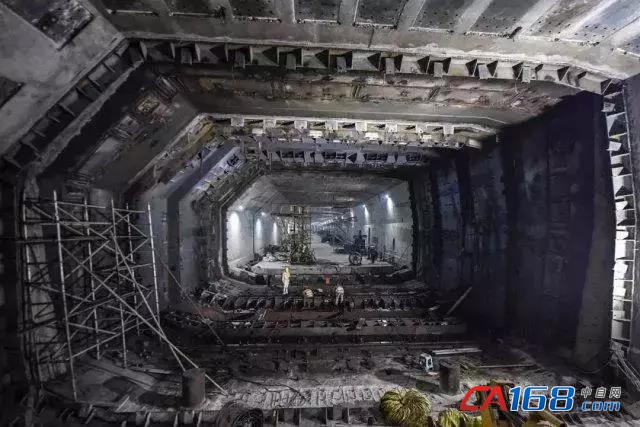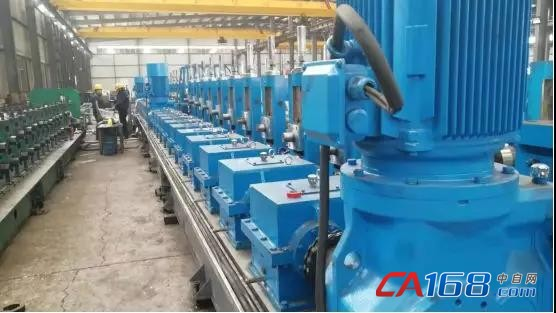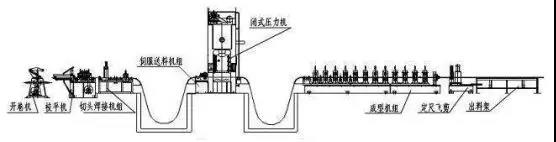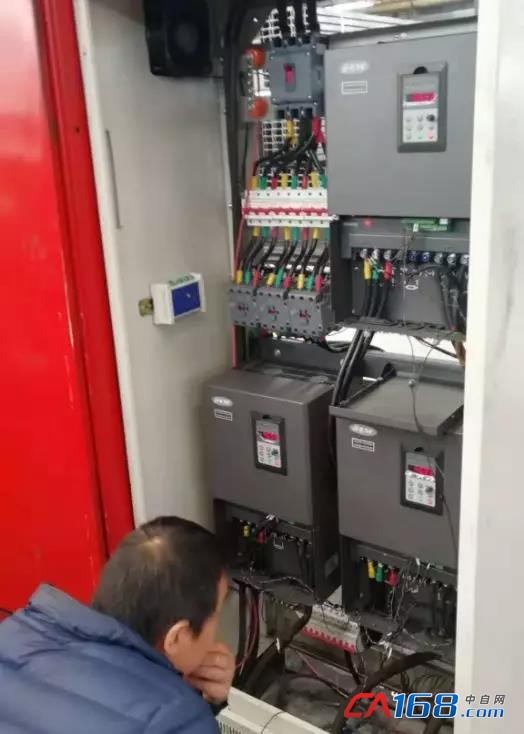（1）自整定设置，F00.24=1，F15.01=37，F15.02=380，F15.03=68，F15.04=50，F15.05=1440，F15.06=2，F15.19=1.设置好后按RUN键进行电机参数静止自整定

（2）主机参数：F00.00=2，F01.00=1，F01.15=1，F02.11=1，F09.35=2，F09.36=17,F19.43=100

（3）从机参数：F00.00=2，F01.15=1，F09.35=2，F09.36=16，F14.00=1，F14.13=002，F14.16=1，F14.17=1，F19.43=100港珠澳大桥的建成意味着国家桥梁技术的更进一步，冷弯型钢机组起到了催化剂的作用，在当前机遇与挑战并存的国际竞争格局中，冷弯钢机组智造转型势在必行，装备强则国强，易能电气愿继续发挥在冷弯性钢机组行业的技术优势，加速推动我国冷弯性钢机组行业崛起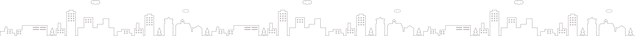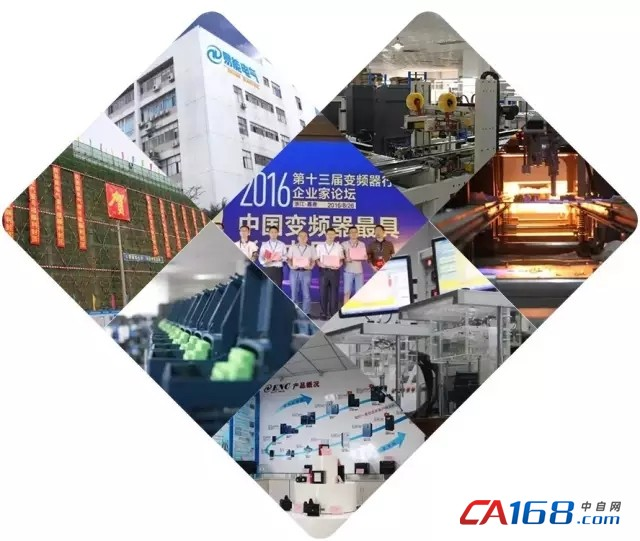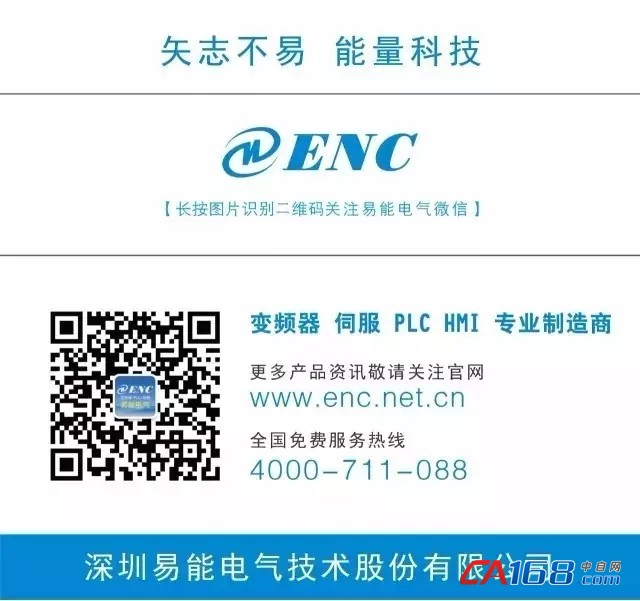[新闻搜索] [] [告诉好友] [打印本文] [关闭窗口] [返回顶部]